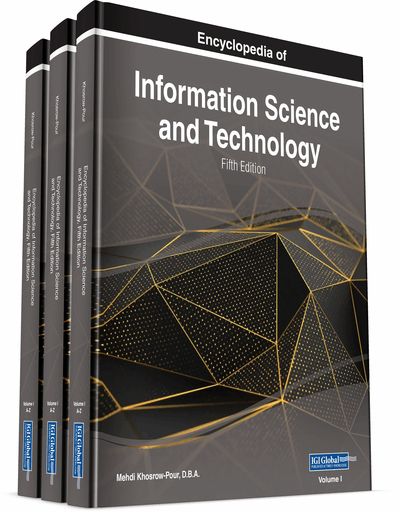# Statistical Techniques for Research

Jose Carlos Casas-Rosal, Carmen León-Mantero, Noelia Jiménez-Fanjul, Alexander Maz-Machado
DOI: 10.4018/978-1-7998-3479-3.ch044
OnDemand:
(Individual Chapters)
Available
\$29.50
No Current Special Offers

## Abstract

Data analysis and statistics are tools that are involved in research and are essential to it. There is a wide variety of techniques that allow one to analyze any set of data depending of the desired goal. However, and due to the variety of techniques, its misuse is very common, and the results obtained from its application cannot be taken into consideration because of its non-validity. The objective of this chapter is to create a classification of the main statistical techniques used in different research fields, adding a brief definition of them and specifying their utility, data hypothesis needed for their use, and software required to use them. Special emphasis is placed in R, a free and open source software with multiple packages what allows one to apply these techniques in an effective and simple way. Among the statistical techniques that are desired to be included in this classification are descriptive analysis, graphic analysis, parametrical and non-parametrical hypothesis testing, principal component analysis, factor analysis, and structural equation modeling.
Chapter Preview
Top

## Background

The word Statistics comes from the Italian word Statista, meaning “Statesman” – referring to its first meaning as a science used by the Government to collect socio-demographic information from its population. From its introduction, it has experimented several extensions in its definition. During the nineteenth century, its field of action was enlarged to the “recollection of information from any data set”, and therefore, its application to other disciplines was extended as well. Later, the analysis and interpretation of data was also considered as a part of the discipline.

However, even though the origin of the word dates from the eighteenth century, Statistics had already been used by the Babylonian and Egyptian civilizations in the elaboration of frequency tables and population censuses. Several authors consider four fundamental periods during the development of Statistics: a period up to 1750 is characterized by the development of probability and the exposition of non-probabilistic methods of data analysis; a period from 1750 to 1820 when inference and mathematical statistics were introduced with works from Laplace and Gauss; a period from 1820 to the early twentieth century with works from Galton, Pearson and Fisher, when statistical inference, correlation and statistical models were developed; and a fourth period, during the last third of the twentieth century, with a strong evolution of Computer Science (Fienberg, 1992). Since then, the amount of techniques developed has been significantly extended due to the calculation capacity of computers.

## Key Terms in this Chapter

Data Analysis: It is a set of techniques that allow us to examine a data set with the aim of obtaining a set of conclusions that can facilitate later decision-making.

Statistical Inference: It is a set of techniques of data analysis that, together with probability calculus, allow to induce the behaviour of a population from the information provided by a sample of it.

Structural Equation Models: Confirmatory technique of data analysis that allows to demonstrate an estimate effects and causal relationships between multiple variables, hidden or observable, through a family of multivariate models.

Causal Models: Mathematical functions that allow estimating the value or one or more variables, called “endogenous variables”, from known values of other variables that have a significant influence on the former and that are called “exogenous variables”. Its construction is done by minimizing the error made during the estimation.

Factory Analysis: Multivariate data analysis technique that allows to reduce the dimensionality of a high set of interrelated variables through a set of variables called “factors”, from which the original variables can be extracted by means of linear combinations of them. Their construction is done by maximizing the common variance of the variables.

Principal Component Analysis: Multivariate data analysis technique that allows to reduce the dimensionality of a high set of interrelated variables through linear combinations ordered from greater to lesser explanatory capacity of the total variance of the set, and independent of each other.

## Complete Chapter List

Search this Book:
Reset